# pi

(redirected from Archimedes constant)
Also found in: Dictionary, Thesaurus, Medical.

## pi

pi, in mathematics, the ratio of the circumference of a circle to its diameter. The symbol for pi is π. The ratio is the same for all circles and is approximately 3.1416. It is of great importance in mathematics not only in the measurement of the circle but also in more advanced mathematics in connection with such topics as continued fractions, logarithms of imaginary numbers, and periodic functions. Throughout the ages progressively more accurate values have been found for π; an early value was the Greek approximation 31-7, found by considering the circle as the limit of a series of regular polygons with an increasing number of sides inscribed in the circle. About the mid-19th cent. its value was figured to 707 decimal places and by the mid-20th cent. an electronic computer had calculated it to 100,000 digits. Although it has now been calculated to some 2.6 trillion digits, the exact value of π cannot be computed. It was shown by the German mathematician Johann Lambert in 1770 that π is irrational and by Ferdinand Lindemann in 1882 that π is transcendental; i.e., cannot be the root of any algebraic equation with rational coefficients. The important connection between π and e, the base of natural logarithms, was found by Leonhard Euler in the famous formula eiπ=−1, where i=√−1.
The following article is from The Great Soviet Encyclopedia (1979). It might be outdated or ideologically biased.

## Pi

π, a letter of the Greek alphabet used in mathematics to denote a particular irrational number—the ratio of the circumference to the diameter of a circle. The symbol was probably adopted from the Greek word for “circumference,” or “periphery.” Although it came into general use after a paper by L. Euler in 1736, it was first used by the British mathematician W. Jones in 1706. Like all irrational numbers, π is an infinite nonrepeating decimal fraction:

π = 3.141592653589793238462643…

The requirements of practical calculations involving circles and circular solids long ago made it necessary to find approximations of π by rational numbers. In the second millennium B.C., ancient Egyptian computations of the area of a circle made use of the approximation π ≈ 3, or, more precisely, π ≈ (16/9)2= 3.16049.… In the third century B.C., Archimedes found, by comparing the circumference of a circle to regular inscribed and circumscribed polygons, that π is between the values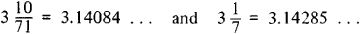The second value is still used in calculations that do not require great accuracy. In the second half of the fifth century, the Chinese mathematician Tsu Ch’ung-chih obtained the approximation 3.1415927, which much later (16th century) was also found in Europe. This approximation is exact for the first six decimal places.

The search for a more exact approximation of π continued in later periods. For example, in the first half of the 15th century, al-Kashi calculated π to 17 places. In the early 17th century, the Dutch mathematician Ludolph van Ceulen obtained 32 places. For practical needs, however, it is sufficient to have values for π and the simplest expressions in which π occurs to only a few decimal places; reference works usually give four- to seven-place approximations for π, 1/π, π2, and log π.

The number π appears not only in the solution of geometric problems. Since the time of F. Vieta (16th century), the limits of certain arithmetic sequences generated by simple rules have been known to involve π. An example is Leibniz’ series (1673-74)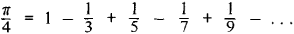This series converges extremely slowly. There exist series for calculating π that converge much more rapidly. An example is the formula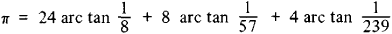where the values of the arc tangents are calculated by means of the series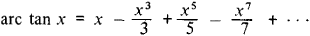The formula was used in 1962 for a computer calculation of π to 100,000 places. This type of calculation is of interest in connection with the concept of random and pseudorandom numbers. Statistical processing has shown that this set of 100,000 digits exhibits many features of a random sequence.

The possibility of a purely analytic definition of π is of fundamental importance for geometry. Thus, in non-Euclidean geometry π also occurs in some formulas but is no longer the ratio of the circumference to the diameter of a circle, for the ratio is not a constant in non-Euclidean geometry. The arithmetic nature of π was finally clarified by analytic means, among which a crucial role was played by the remarkable Euler formula e2πi = 1, where e is the base of the natural system of logarithms and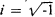.

At the end of the 18th century, J. H. Lambert and A. M. Legendre proved that π is irrational. In 1882 the German mathematician F. Lindemann showed it to be transcendental—that is, it cannot satisfy any algebraic equation with integral coefficients. The Lindemann theorem conclusively established that the problem of squaring the circle cannot be solved by means of a compass and straightedge.

### REFERENCES

O kvadrature kruga (Arkhimed, Giuigens, Lambert, Lezhandr): Sprilozheniem istorii voprosa…, 3rd ed. Moscow-Leningrad, 1936. (Translated from German.)
Shanks, D., and J. W. Wrench. “Calculation of π to 100,000 Decimals.” Mathematics of Computation, 1962, vol. 16, no. 77.

## pi

[]
(mathematics)
The irrational number which is the ratio of the circumference of any circle to its diameter; an approximation is 3.14159. Symbolized π.
McGraw-Hill Dictionary of Scientific & Technical Terms, 6E, Copyright © 2003 by The McGraw-Hill Companies, Inc.

## pi

1. the 16th letter in the Greek alphabet (Π, π), a consonant, transliterated as p
2. Maths a transcendental number, fundamental to mathematics, that is the ratio of the circumference of a circle to its diameter. Approximate value: 3.141 592…; symbol: π
Collins Discovery Encyclopedia, 1st edition © HarperCollins Publishers 2005

## PI

An interface between Prolog application programs and the X Window System that aims to be independent from the Prolog engine, provided that it has a Quintus foreign function interface (e.g. SICStus and YAP). It is mostly written in Prolog and is divided in two libraries: Edipo - the lower level interface to the Xlib functions; and Ytoolkit - the higher level user interface toolkit.

ftp://ftp.ncc.up.pt/pub/prolog/ytoolkit.tar.Z.

E-mail: Ze' Paulo Leal <zp@ncc.up.pt>.

## pi

(1) See Raspberry Pi.

(2) The 16th letter of the Greek alphabet. It is used as a symbol for the ratio of the circumference of a circle to its diameter, which is 3.141592653, commonly rounded to 3.14. Perhaps no other number has been more pondered, examined and calculated than pi. Circa 1650 B.C., the ratio was computed by an Egyptian scribe, and the number was recorded as 3.16049 in the Rhind Papyrus. The writings described how to create a square area the same size as a circle.

The Exact Value of Pi
Over the years, pi, which was named some 3,000 years later, has been calculated numerous times to the maximum decimal place that humans and calculating devices could take it. In 1596, it was calculated to 32 decimal places and up to 127 places by 1719. In 1949, the ENIAC took 70 hours to yield 2,037 digits. However, in 1997, a Hitachi mainframe computed pi to 51.5 billion digits in 29 hours. The bottom line is that the absolutely exact value of pi cannot be computed.

The Pi SymbolCopyright © 1981-2019 by The Computer Language Company Inc. All Rights reserved. THIS DEFINITION IS FOR PERSONAL USE ONLY. All other reproduction is strictly prohibited without permission from the publisher.
Site: Follow: Share:
Open / Close